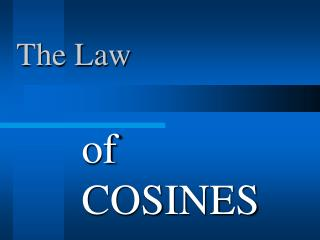DownloadDownload PresentationThe Law

# The Law

Télécharger la présentation## The Law

- - - - - - - - - - - - - - - - - - - - - - - - - - - E N D - - - - - - - - - - - - - - - - - - - - - - - - - - -
##### Presentation Transcript

1. The Law of COSINES

2. The Law of COSINES For any triangle (right, acute or obtuse), you may use the following formula to solve for missing sides or angles:

3. Use Law of COSINES when ... • SAS- 2 sides and their included angle • SSS you have 3 dimensions of a triangle and you need to find the other 3 dimensions . They cannot be just ANY 3 dimensions though, or you won’t have enough information to solve the Law of Cosines equations. Use the Law of Cosines if you are given:

4. B 98° c = 20 a = 13 C A b Example 1: Given SAS Find all the missing dimensions of triangle ABC, given that angle B = 98°, side a = 13 and side c = 20. Use the Law of Cosines equation that uses a, c and B to find side b:

5. B 98° c = 20 a = 13 C A b = 25.3 Example 1: Given SAS Now that we know B and b, we can use the Law of Sines to find one of the missing angles: Solution: b = 25.3, C = 51.5°, A = 30.5°

6. B c = 15 a 39° A C b = 20 Example 2: Given SAS Find all the missing dimensions of triangle, ABC, given that angle A = 39°, side b = 20 and side c = 15. Use the Law of Cosines equation that uses b, c and A to find side a:

7. a = 12.6 B c = 15 39° A C b = 20 Example 2: Given SAS Use the Law of Sines to find one of the missing angles: Important: Notice that we used the Law of Sine equation to find angle C rather than angle B. The Law of Sine equation will never produce an obtuse angle. If we had used the Law of Sine equation to find angle B we would have gotten 87.5°, which is not correct, it is the reference angle for the correct answer, 92.5°. If an angle might be obtuse, never use the Law of Sine equation to find it.

8. A c = 15 b = 20 C B a = 30 Example 3: Given SSS Find all the missing dimensions of triangle, ABC, given that side a = 30, side b = 20 and side c = 15. We can use any of the Law of Cosine equations, filling in a, b & c and solving for one angle. Once we have an angle, we can either use another Law of Cosine equation to find another angle, or use the Law of Sines to find another angle.

9. A c = 15 b = 20 C B a = 30 Example 3: Given SSS Important: The Law of Sines will never produce an obtuse angle. If an angle might be obtuse, never use the Law of Sines to find it. For this reason, we will use the Law of Cosines to find the largest angle first (in case it happens to be obtuse). Angle A is largest because side a is largest:

10. 117.3° A c = 15 b = 20 C B a = 30 Example 3: Given SSS Use Law of Sines to find angle B or C (its safe because they cannot be obtuse): Solution: A = 117.3° B = 36.3° C = 26.4°

11. When given one of these dimension combinations, use the Law of Cosines to find one missing dimension and then use Law of Sines to find the rest. • SAS • SSS The Law of Cosines Important: The Law of Sines will never produce an obtuse angle. If an angle might be obtuse, never use the Law of Sines to find it.